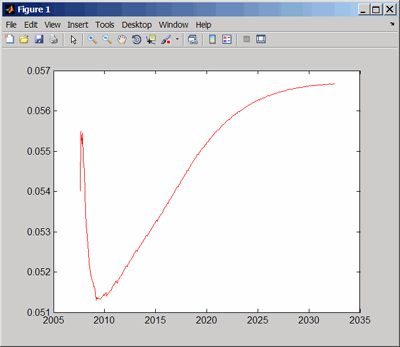# Yield Curve

## Build, plot, and analyze the yield curve

A yield curve is a graph that plots interest rates or yields of similar fixed-income instruments with differing maturities across time. The curve creates a visual representation of the term structure of interest rates. By aggregating lender priorities over time for a particular borrower or credit risk profile, yield curves enable you to study financial market conditions and analyze potential investments or trading opportunities.Plotting a yield curve in MATLAB.

Yield curves are borrower-specific, so different curves are constructed for sovereign debt (e.g., the US Treasury default-free curve), the interbank markets (the swap curve), and corporate debt (a credit spread over the swap curve).

They are typically constructed and calibrated to the market prices of a variety of fixed-income instruments, including government debt, money market rates, short-term interest rate futures, and interest rate swaps. To build a smooth and consistent curve, you use a combination of bootstrapping, curve fitting, and interpolation techniques. These curves, once constructed, can then be used to price other OTC derivatives consistently with the markets.

For more information, see MATLAB® toolboxes for finance, data feeds, financial instruments, statistics, and curve fitting.

### Software Reference

See also: financial engineering, fixed income, financial derivatives, swap curve, zero curve, Econometrics Toolbox, Parallel Computing Toolbox, Symbolic Math Toolbox, Curve Fitting Toolbox, Spreadsheet Link (for Microsoft Excel)